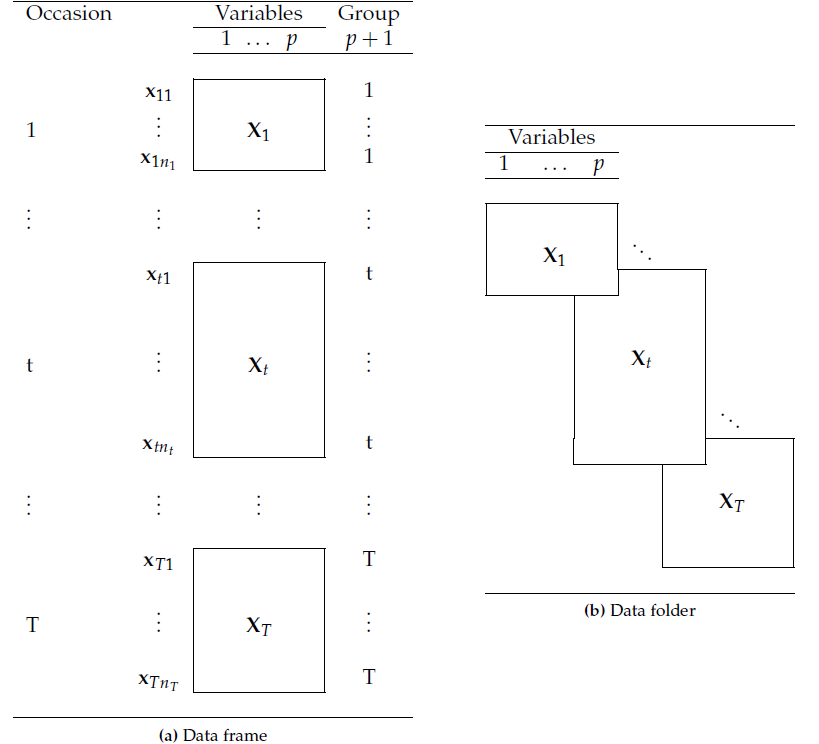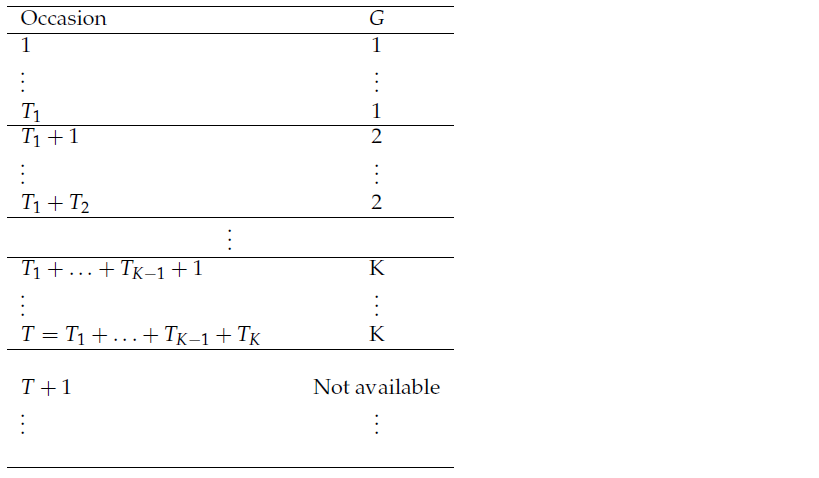## Data under consideration

For the analyses implemented in the dad package, the data $$\mathbf{X}$$ (Table 1) of interest have three kinds of objects: occasions (or groups) $$\times$$ individuals $$\times$$ variables. In what follows, the terms occasion and group are used interchangeably: in the case of three-way data, the term occasion would be preferable, while in the case of multigroup data the term group would be more appropriate.

The groups define a partition of the individuals on which are measured the variables. If $$T$$ denotes the number of groups, for each $$t$$ in $$\left\{1,\ldots,T\right\}$$ the rows of the table $$\mathbf{X}_t$$ correspond to $$n_t$$ observations $$\mathbf{x}_{t1}\,,\ldots,\,\mathbf{x}_{tn_t}$$ of $$X_t$$ a random vector with $$p$$ components.Table 1: For each group (or occasion) $$t = 1,\ldots,T$$, the same $$p$$ variables are observed for $$n_t$$ individuals.

For discriminant analysis, the data of interest are similar to the previous ones with the difference that we have two categories of occasions or groups. The first category, consisting of $$T$$ occasions, are partitioned into $$K$$ subsets deriving from a factor $$G$$ defined on occasions . The second category consists of occasions, numbered $$T+1,\ \ldots$$ for which we have data of type $$\mathbf{X}$$ but not the value of $$G$$.Table 2: Each occasion $$t$$ ($$t=1,\ldots,T$$) matches a table with $$n_t$$ rows and $$p$$ columns (see Table 1). The variable $$G$$ defined on the occasions takes values $$\{1,\ldots,K\}$$. For each $$k=1,\ldots,K$$, the value $$k$$ is taken $$T_k$$ times. The $$G$$ values of the occasions $$T+1,\,\ldots$$ are not available and have to be predicted.

## Implemented methods and their objectives

When the individuals are organised into groups, the analyst could be interested in taking into account this data organisation by associating with each group a mathematical object and performing multivariate techniques on these objects. In the dad package devoted to such data, the objects are probability density functions. These densities are either all continuous (numeric data with Lebesgue measure as reference measure) or all discrete (categorical data with counting measure as reference measure) and are subjected to following analyses:

• Multidimensional scaling (MDS) of probability density functions aims to visualize a set of densities (or groups) so that the distances between the densities are preserved as well as possible;
• Hierarchical cluster analysis (HCA) of probability density functions is used to divide a set of densities (or groups) into clusters so that the densities of the same cluster are as similar as possible, and are dissimilar from those of the other clusters;
• Discriminant analysis (DA) of probability density functions deals with the same kind of data, knowing a partition of the densities (or groups) into classes. Its first objective is to learn how the a priori classes can be explained by the distances between these densities. Then if the training step is judged satisfactory according to a criterion named misclassification ratio, its second objective is to classify a new density whose class is unknown.

These three multivariate techniques are essentially based on distance indices between probability density functions. Literature abounds with such indices: as an example, the encyclopedia of distances of Deza (p. 235–245)1 lists some forty. The package proposes to calculate ten of them by considering the case of discrete densities and that of continuous densities. The results returned by the three previous multivariate techniques depend on the distance index used. The choice of such a distance index depends above all on the modeling hypotheses: discrete or continuous data, Gaussian or not…

Thus, for each distance index, the dad package implements:

• its calculation for two densities whose type and parameters are known,
• its estimation for two densities for which there are two samples which allow the estimation of their parameters,
• the generalization of each previous calculation for $$T$$ ($$T > 2$$) densities taken two by two, the result of which is a symmetric matrix.

## Data organisation

The dad package uses objects of class "folder" or "folderh". These objects are lists of data frames having particular formats.

### Objects of class folder

Such objects are lists of data frames which have the same column names. They are created by the functions folder or as.folder (see their help in R).

data("roses")
rosesf <- as.folder(roses[,c("Sha", "Den", "Sym", "rose")], groups = "rose")
print(rosesf, max = 9)
## $A ## Sha Den Sym ## 1 7.0 6.7 6.7 ## 2 7.1 7.8 8.1 ## 3 7.0 6.8 7.4 ## [ reached 'max' / getOption("max.print") -- omitted 39 rows ] ## ##$B
##    Sha Den Sym
## 43 8.1 7.7 3.0
## 44 8.6 5.9 6.7
## 45 7.7 6.7 7.4
##  [ reached 'max' / getOption("max.print") -- omitted 39 rows ]
##
## $C ## Sha Den Sym ## 85 0.7 9.3 1.4 ## 86 2.3 7.7 2.4 ## 87 3.6 7.9 7.2 ## [ reached 'max' / getOption("max.print") -- omitted 39 rows ] ## ##$D
##     Sha Den Sym
## 127 9.2 1.8 9.0
## 128 9.0 2.3 9.2
## 129 6.9 2.6 7.6
##  [ reached 'max' / getOption("max.print") -- omitted 39 rows ]
##
## $E ## Sha Den Sym ## 169 5.6 1.7 8.2 ## 170 7.5 3.4 8.6 ## 171 5.8 3.9 5.8 ## [ reached 'max' / getOption("max.print") -- omitted 39 rows ] ## ##$F
##     Sha Den Sym
## 211 8.3 8.0 6.5
## 212 8.4 7.8 3.3
## 213 9.2 8.2 7.6
##  [ reached 'max' / getOption("max.print") -- omitted 39 rows ]
##
## $G ## Sha Den Sym ## 253 8.6 2.0 5.4 ## 254 8.5 2.3 7.9 ## 255 7.6 3.5 7.1 ## [ reached 'max' / getOption("max.print") -- omitted 39 rows ] ## ##$H
##     Sha Den Sym
## 295 6.5 4.3 2.6
## 296 6.6 2.9 2.9
## 297 8.4 5.1 6.4
##  [ reached 'max' / getOption("max.print") -- omitted 39 rows ]
##
## $I ## Sha Den Sym ## 337 4.9 6.5 7.6 ## 338 5.8 6.6 7.9 ## 339 4.3 5.6 6.0 ## [ reached 'max' / getOption("max.print") -- omitted 39 rows ] ## ##$J
##     Sha Den Sym
## 379 4.9 5.2 8.9
## 380 4.6 8.1 8.6
## 381 3.5 7.8 7.4
##  [ reached 'max' / getOption("max.print") -- omitted 39 rows ]
##
## attr(,"class")
##  "folder"
## attr(,"same.rows")
##  FALSE

### Objects of class folderh

In the most useful case, such objects are hierarchical lists of two data frames df1 and df2 related by means of a key which describes the “1 to N” relationship between the data frames. They are created by the function folderh (see its help in R for the case of three data frames or more).

data(roseflowers)
df1 <- roseflowers$variety df2 <- roseflowers$flower
fh1 <- folderh(df1, "rose", df2)
print(fh1)
## $df1 ## place rose variety ## 34 outdoors 34 v1 ## 40 outdoors 40 v4 ## 60 outdoors 60 v3 ## 66 glasshouse 66 v3 ## 68 glasshouse 68 v4 ## ##$df2
##    rose numflower diameter height nleaves
## 1    34         1     94.5   57.0       8
## 2    34         2     89.5   54.0      10
## 3    40         1     57.0   21.5       9
## 4    40         2     52.5   20.5       5
## 5    40         3     51.5   14.0       7
## 6    60         1     53.0   23.0       4
## 7    60         2     52.0   24.5       9
## 8    66         1     35.0    9.5       4
## 9    66         2     35.0   14.0       6
## 10   66         3     36.0   13.5       7
## 11   68         1     45.5   19.5      10
##
## attr(,"class")
##  "folderh"
## attr(,"keys")
##  "rose"

1. Deza, M.M. and Deza, E. (2013), Encyclopedia of Distances. Springer-Verlag, Heidelberg.↩︎